Previous: 8.3.1 Continuity Equation Up: 8.3 Conservation of Mass Next: 8.4 Discretization

## 8.3.2 Meshing Constraints

Conservation of mass is the one most important property a discretization of the Wigner equation has to fulfill. This subsection discusses the constraints this imposes on the mesh.

The main difficulty in achieving a discrete conservation law lies in the discretization of the drift term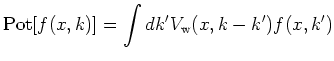We write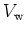in the form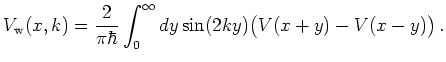(8.9)

We need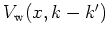for every pair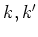. For a general mesh one getsdifferent values for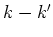and needs as many evaluations of the integral 8.9. Hence only from feasibility requirements alone one is in practice often forced to use an equispaced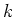-grid.

In the continuous infinite case the-integral over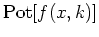vanishes becauseis odd inand the integral appearing on the right hand side of Equation 8.7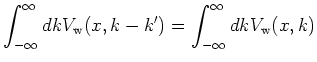(8.10)

extends over the whole-space.

To discuss the discrete case we assume that the-domain used for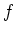has limits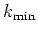and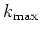. We denote the length of the integration domain by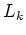.

Then the integration argumentextends fromtoand we integrate over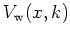with limits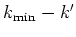to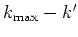in the right hand side of Equation 8.10.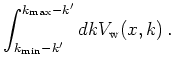(8.11)

Now the integration interval is symmetric around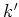(and not around 0) and in general the integral no longer vanishes, even ifis an odd function in.

The integral 8.11 vanishes in the case thatis not only odd but also periodic with period. Then the integral 8.11 always extends over exactly one period and hence vanishes in each case as it is independent of.

To achieve a periodicin the case of discrete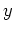one can introduce a special mesh. This method was introduced by Frensley [Fre90]. It assumes an arbitrary (possibly shifted) equispaced mesh forin 8.9. Here we write the-mesh in the unshifted form(8.12)

The condition for an integrand which is periodic inin Equation 8.9 is given as(8.13)

This condition is fulfilled if the phase difference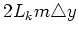(8.14)

is a multiple of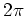. From this we derive the condition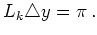(8.15)

This condition is known from [Fre90] as a completeness condition. It links the spacing of the-mesh in the calculation of the Wigner potential with an apparent periode lengthof.

We now assume thatis odd inand periodic with period. Then in order that the discrete version of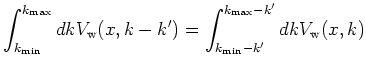vanishes (withfrom the-mesh for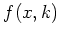) we can choose an equispaced-mesh satisfying the constraint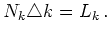(8.16)

The meshing condition 8.15 fixes only the spacing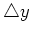to be used in the discrete form of 8.9 but it does not fix the limits of the integral, i.e., the number of points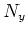. However, given an equispaced-mesh forwith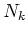points, it seems natural to choose the mesh in such a way that definition 8.9 corresponds to the expansion of an odd function into a series of sines. Then the denomination completeness'' is also justified. The discrete sine transform is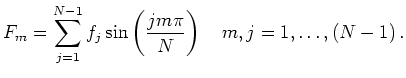(8.17)

Comparing with 8.9 and using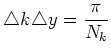(8.18)

we get the discrete form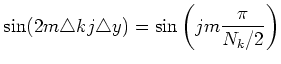(8.19)

and the discrete form of 8.9 finally becomes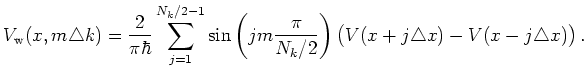(8.20)

The-mesh has an even number of pointswhich we choose symmetric around zero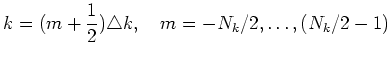We denote the quantity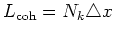(8.21)

as the coherence length. It is introduced here for purely numerical reasons but also allows for a physical interpretation.

We want to stress, that the-mesh used in the discretization of the Wigner potential can in principle be chosen independently and differently from the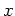-mesh, especially, if the band is given analytically - for example, in a flat band model. In a self-consistent simulation one normally uses the same mesh forand for. In any case the numberof points inand the number of points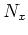incan be chosen independently and usually one choosesslightly smaller than.

In practice one starts with choosing an equispaced mesh usingpoints for the simulation domain. Then one chooses a coherence lengthwhich is a multiple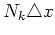of the-mesh spacing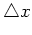, see Equation 8.21. The period lengthof the-mesh is then fixed by the completeness condition Equation 8.15 as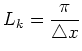(8.22)

where we used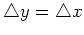. Finally, the-spacing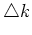is given as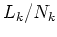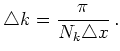(8.23)

The artificial introduction of a Wigner potentialwhich is periodic inis an elegant trick to obtain a discretization conserving mass. We also experimented with meshes which do not obey this completeness condition. But the results from such simulations were unusable for TCAD purposes. Hence meshing is essentially restricted to the use of an equispaced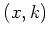-product grid.

These discussions are in accordance with transformation-theoretic theorems stating that in a certain sense there are no reasonable discretizations of the Wigner transform. Every discretization lacks one or more properties characteristic of the continuous transform.